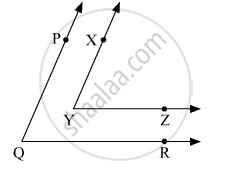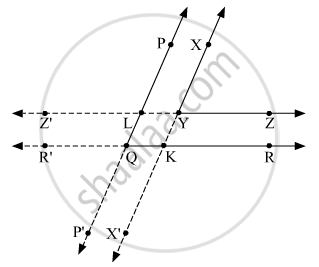Advertisement Remove all ads

# In the Given Figure, Sides of ∠ Pqr and ∠ Xyz Are Parallel to Each Other.Prove That, ∠ Pqr ≅ ∠ Xyz - Geometry

Sum

In the given figure, sides of angle PQR and angle XYZ are parallel to each other.Prove that, angle PQR  ≅ angle XYZ.

Advertisement Remove all ads

#### SolutionWe have, XY || PQ and YZ || QR.
Let us produce rays YZ and YX backwards to point Z' and X' respectively such that ZYZ' and XYX' are the straight lines.
Similarly, produce the rays QP and QR to points P' and R' respectively such that PQP' and RQR' are straight lines.
Suppose line ZYZ' intersects the line PQP' at L and line RQR' intersects the line XYX' at K.
Now, line XYX' || line PQP' and line ZYZ' || line RQR'.

Since, line ZYZ' || line RQR' and line XYX' is a transversal intersecting them at Y and K, then
m∠XYZ = m∠XKR    (Corresponding angles)      ....(1)
Since, line XYX' || line PQP' and line RQR' is a transversal intersecting them at Q and K, then
m∠PQR = m∠XKR    (Corresponding angles)      ....(2)
From (1) and (2), we get
m∠XYZ = m∠PQR
⇒∠XYZ ≅ ∠PQR.

Is there an error in this question or solution?
Advertisement Remove all ads

#### APPEARS IN

Balbharati Mathematics 2 Geometry 9th Standard Maharashtra State Board
Chapter 2 Parallel Lines
Practice Set 2.1 | Q 4 | Page 17
Advertisement Remove all ads
Advertisement Remove all ads
Share
Notifications

View all notifications

Forgot password?